# How to work out fractions of numbers

Want to find out how to work out fractions of numbers? We've provided a step-by-step guide of how to work out fractions of numbers to teach you everything you need to know.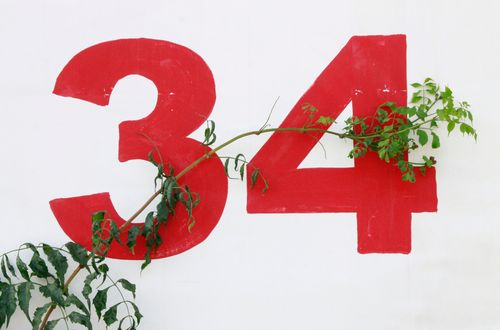## Step 1: Convert to a mixed fraction

If the fraction is a mixed fraction, change first to its mixed fraction. If otherwise, proceed to the next step. For example, if we want to find 1 1/2 of 48, we’ll need to change 1 1/2 to improper fraction first.

## Step 2: Multiply by the fraction

To find the fraction of a number, multiply the number by the given fraction. So, we multiply 48 by 3/2.

## Step 3: Cancel out common factors

Cancel out common factors shared by the denominator and numerator.

## Step 4: Multiply the remaining factors

Once all common factors are cancelled out, multiply the remaining factors to find the final result. This means that 1 1/2 of 48 is equal to 72.

## Examples of how to work out fractions of numbers

Q1) Determine the value of the following.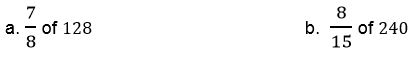To find the values being asked, multiply the numbers by the given fractions.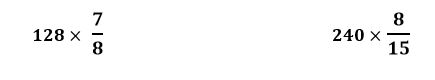We can now look for common factors shared by the numerator and the denominator and cancel these common factors out.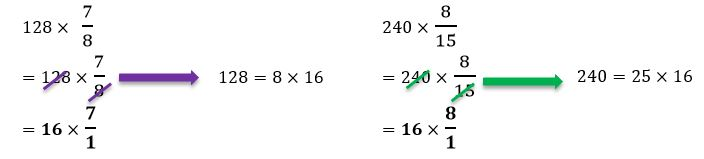Multiply the remaining factors to find their respective products.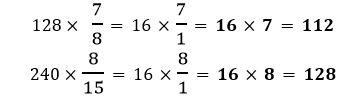Hence, we have the following values.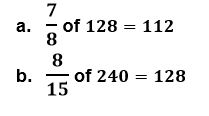Q2) Marissa bought 144 pieces of eggs for her pastry shop’s stock. She’s planning to bake two batches of cupcakes and will be using 5/12 of the shop’s egg stock. How many eggs will Marissa need?

We want to determine the number of eggs that will be used if Marissa needs 5/12 out of 144 eggs.

We multiply 5/12 by 144. Cancel out common factors shared by the numerators and denominators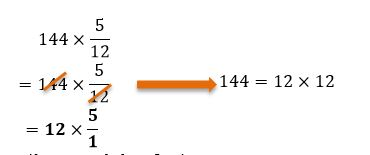Simplify the product by multiplying the remaining factors.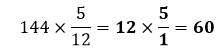This means that Marissa needs 60 eggs to bake two batches of cupcakes.

Q3) Nate was rewarded with \$550 by his parents for performing well in his previous assessment exam. He decided to use 3/10 of this to purchase a new fitness device. How much does the fitness device cost?

To find the cost of the fitness device, we multiply 3/10 by \$550. Simplify the fractions by cancelling out common factors shared by the denominator and numerator.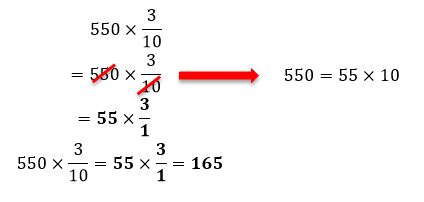Nate bought a fitness device that costs \$165.

#### Join today

The fastest way to practice

Unlock our complete testing platform and improve faster that ever.